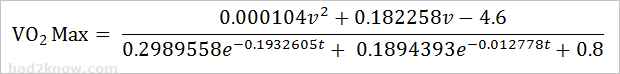# VO2 Max Race Time Prediction Formula and Calculator

Race Time Prediction Calculator
Previous Distance Run meters
Previous Time h
m
s
New Distance to Be Run meters

Runners can estimate their time to finish a race based on the results of a previous race and one of several prediction formulas. One of the preferred methods is based on VO₂ max. A runner's VO₂ max level is more or less constant over short periods of time, and it can be estimated from the results of a previous race using the Daniels and Gilbert equation. Thus, given the values of VO₂ max and the distance of new race to be run, a runner can predict his time to finish the new race.

To apply the Daniels and Gilbert equation, you must convert all distances into meters. For convenience:

1 mile = 1609.344 meters
1 km = 1000 meters

You can also use the length conversion calculator. The Daniels and Gilbert VO₂ formula is explained below. Since the equation cannot be algebraically rearranged to isolate time, one must use numerical algorithms to solve for time.

### The Daniels and Gilbert VO2 Max Formula

Given a race length of d meters, a time of t minutes, and a running speed of v = d/t meters per minute, the Daniels and Gilbert model estimates VO₂ max with the equationAs you can see, the numerator is a rational function of t, while the denominator is an exponential function of t, thus it is not possible to solve for t using simple algebra. The calculator above solves this equation numerically rounding down to the nearest whole second.

Example: Last week, Tom ran 3.5 miles in 51 minutes and 30 seconds, which is equivalent to 5632.704 meters in 51.5 minutes. The week before, he ran 2.5 miles in 34 minutes and 45 seconds, which is equivalent to 4023.36 meters in 34.75 minutes.

He wants to use this information to predict the time it takes to run a 5-mile race, equivalent to 8046.72 meters.

If we estimate his VO₂ max and compute his projected time with the first set of race results, we get

VO2 Max = 18.46 mL/kg/min.               Time = 1h 15m 9s

If we estimate his VO₂ max and compute his projected time with the second set of race results, we get

VO2 Max = 19.41 mL/kg/min.               Time = 1h 12m 14s

The average of these two times is 1h 13m 41.5s. Notice that this is very close to the estimates produced with the Riegel and Cameron formulas.